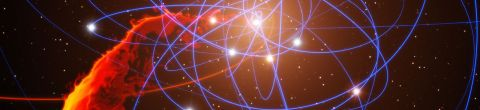CosmologyWinter, 2013

This course of the Theoretical Minimum series will concentrate on cosmology, the science of the origin and development of the universe. Along the way, students will take a close look at the Big Bang, the geometry of space-time, inflationary cosmology, cosmic microwave background, dark matter, dark energy, the anthropic principle, and the string theory landscape. (Image credit: ESO/MPE/Marc Schartmann)

Lectures in this Course

1. The expanding (Newtonian) universe

Professor Susskind introduces the topic of modern Cosmology, which started with the discovery of cosmic microwave background radiation in 1964.  However, this lecture focuses on the classical or Newtonian view of the universe.  Beginning with the... [more]
2. Matter and radiation dominated universes

After reviewing the basic equation for an expanding universe, Professor Susskind solves the equation explicitly for a zero energy universe, and then extends the derivation to universes with non-zero energy.  These universes can take two forms:... [more]
3. Geometries of space: flat, spherical, hyperbolic

Professor Susskind presents three possible geometries of homogeneous space: flat (infinite), spherical (positively curved and finite), hyperbolic (negatively curved and infinite). He develops the metric for these three spatial geometries in... [more]
4. Cosmological thermodynamics

The time-time component of Einstein's field equations for general relativity relate energy density to the geometry of space.  Using this energy density, Professor Susskind presents the thermodynamic equation of state which relates the energy density... [more]
5. Vacuum energy

After a review of the equations of state presented in the last lecture, Professor Susskind derives the density parameter for an energy dominated universe.  He then introduces the concept of vacuum energy, which is represented by the cosmological... [more]
6. Dark matter and allocation of energy density

Professor Susskind develops the energy density allocation equation, and describes the historical progress of the effort to find the correct values for the terms in this equation.  This analysis combined with observations of the motion of stars and... [more]
7. Temperature history of the universe

Professor Susskind examines the temperature history of our universe.  The universe switched from radiation-dominated to matter-dominated when it was about one million times hotter than it is today.  At that time, matter and energy decoupled from... [more]
8. Baryogenesis

Professor Susskind opens the lecture with one of the fundamental questions in cosmology: why are there more protons than anti-protons in the universe today? The answer lies in theory of baryogenesis in the very early universe.  This theory leads to... [more]
9. Inflation

Professor Susskind introduces the theory of cosmological inflation under which the early universe underwent an exponential expansion during which it doubled in size every 10-32 seconds and expanded by at least a factor of e90... [more]
10. Inhomogeneities and quantum fluctuations

Professor Susskind describes the theory whereby inhomogeneities in the universe were caused by quantum fluctuations in the early universe.  He begins with the mathematical equations of a damped harmonic oscillator, and relates that simple system to... [more]